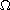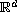Variational and Topological Methods: Theory, Applications, Numerical Simulations, and Open Problems.
Electron. J. Diff. Eqns., Conference 21 (2014), pp. 61-76.

### Symmetry analysis and numerical solutions for semilinear elliptic systems C. Tyler Diggans, John M. Neuberger, James W. Swift

Abstract:
We study a two-parameter family of so-called Hamiltonian systems defined on a regioninwith the bifurcation parametersandof the form:takingto be a function of two variables satisfying certain conditions. We use numerical methods adapted from Automated Bifurcation Analysis for Nonlinear Elliptic Partial Difference Equations on Graphs (Inter. J. Bif. Chaos, 2009) to approximate solution pairs. After providing a symmetry analysis of the solution space of pairs of functions defined on the unit square, we numerically approximate bifurcation surfaces over the two dimensional parameter space. A cusp catastrophe is found on the diagonal in the parameter space whereand is explained in terms of symmetry breaking bifurcation. Finally, we suggest a more theoretical direction for our future work on this topic.

Published February 10, 2014.
Math Subject Classifications: 35J15, 65N30.
Key Words: Nonlinear elliptic PDE; elliptic systems; Newton's method; GNGA; bifurcation.

Show me the PDF(920 K), TEX and other files for this article.C. Tyler Diggans Department of Mathematics Northern Arizona University, 86005 Flagstaff, AZ 86011, USA email: Tyler.Diggans@nau.edu John M. Neuberger Department of Mathematics Northern Arizona University, 86005 Flagstaff, AZ 86011, USA email: John.Neuberger@nau.edu Jim W. Swift Department of Mathematics Northern Arizona University, 86005 Flagstaff, Arizona, USA email: Jim.Swift@nau.edu